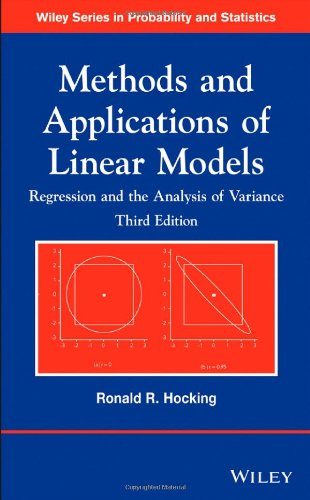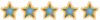Boxutech
Welcome to Boxutech
Boxutech
Would you like to react to this message? Create an account in a few clicks or log in to continue.

# Methods and Applications of Linear Models: Regression and the Analysis of Variance (Wiley Series in Probability and Statistics)##Methods and Applications of Linear Models: Regression and the Analysis of Variance (Wiley Series in Probability and Statistics)A thoroughly updated book, Methods and Applications of Linear Models: Regression and the Analysis of Variance, Third Edition features innovative approaches to understanding and working with models and theory of linear regression. The Third Edition provides readers with the necessary theoretical concepts, which are presented using intuitive ideas rather than complicated proofs, to describe the inference that is appropriate for the methods being discussed.

The book presents a unique discussion that combines coverage of mathematical theory of linear models with analysis of variance models, providing readers with a comprehensive understanding of both the theoretical and technical aspects of linear models. With a new focus on fixed effects models, Methods and Applications of Linear Models: Regression and the Analysis of Variance, Third Edition also features:

Newly added topics including least squares, the cell means model, and graphical inspection of data in the AVE method
Frequent conceptual and numerical examples for clarifying the statistical analyses and demonstrating potential pitfalls
Graphics and computations developed using JMP® software to accompany the concepts and techniques presented
Numerous exercises presented to test readers and deepen their understanding of the material

An ideal book for courses on linear models and linear regression at the undergraduate and graduate levels, the Third Edition of Methods and Applications of Linear Models: Regression and the Analysis of Variance is also a valuable reference for applied statisticians and researchers who utilize linear model methodology.

Product Details

Series: Wiley Series in Probability and Statistics
Hardcover: 720 pages
Publisher: Wiley; 3 edition (July 29, 2013)
Language: English
ISBN-10: 1118329503
ISBN-13: 978-1118329504bacsikha